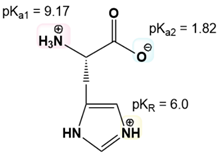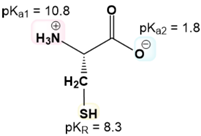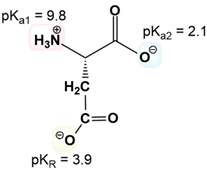Clutch Prep is now a part of Pearson
Ch. 3 - Amino AcidsWorksheetSee all chapters

# Isoelectric Point of Amino Acids with Ionizable R-Groups

See all sections
Sections
Amino Acid Groups
Amino Acid Three Letter Code
Amino Acid One Letter Code
Amino Acid Configuration
Essential Amino Acids
Nonpolar Amino Acids
Aromatic Amino Acids
Polar Amino Acids
Charged Amino Acids
How to Memorize Amino Acids
Zwitterion
Non-Ionizable Vs. Ionizable R-Groups
Isoelectric Point
Isoelectric Point of Amino Acids with Ionizable R-Groups
Titrations of Amino Acids with Non-Ionizable R-Groups
Titrations of Amino Acids with Ionizable R-Groups
Amino Acids and Henderson-Hasselbalch

Concept #1: Calculating Isoelectric Point Of Amino Acids With Ionizable R-Groups

Practice: What is the pI of His?Practice: Electrophoresis separates molecules by migration through a gel only if they have a net charge. How do you expect an amino acid to migrate during electrophoresis when the pH = pI?

Practice: Draw Glu and calculate its isoelectric point. pKa1 = 9.67. pKa2 = 2.19. pKR = 4.25.

Practice: Calculate Cys’s pI.Practice: Calculate the pI of Arg. pKa1 = 9. pKa2 = 2.2. pKR = 12.5.

Practice: Calculate the pI of Asp.